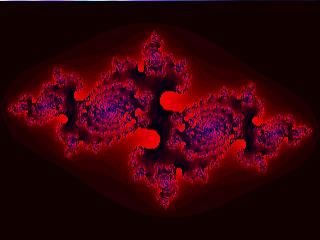DownloadDownload PresentationChaotic Mining: Knowledge Discovery Using the Fractal Dimension

# Chaotic Mining: Knowledge Discovery Using the Fractal Dimension

Télécharger la présentation## Chaotic Mining: Knowledge Discovery Using the Fractal Dimension

- - - - - - - - - - - - - - - - - - - - - - - - - - - E N D - - - - - - - - - - - - - - - - - - - - - - - - - - -
##### Presentation Transcript

1. Chaotic Mining: Knowledge Discovery Using the Fractal Dimension Daniel Barbara George Mason University Information and Software Engineering Department dbarbara@gmu.edu By Dhruva Gopal

2. Fractals • What are fractals • Property of a fractal • Self Similarity

3. Uses of fractals • Geologic activity • Planetary orbits • Weather • Fluid flow • databases

4. Fractal Dimensions • Number of possible dimensions? • Fractal dimension computation • Dq = 1/(q-1)*(logSipiq)/(log r) • Hausdorff dimension • Information dimension • Correlation dimension

5. Examples • Event Anomalies in time series • Self similarity in association rules • Analyzing patterns in datacubes • Incremental clustering

6. Event Anomalies • Time series • Stock price changes • TCP connection occurrence • Example • Half open TCP connections • Network Spoofing

7. Methodology • Half open connections are self similar • Collect data points every d seconds • Moving window of k * d (k is an integer) • Fractal dimension will show a drastic decrease in case of spoofing • Other applications of fractals with time series • Password port in FTP service

8. Self Similarity in Association Rules • Parameters associated with a rule • Support • Confidence • Distribution of these transactions??? • Seasonal • Promotional • Regular

9. Fractals in Association rules • Compute Fractal dimension of a k-itemset while computing its support • Information about the fractal dimension should be kept for use when computing k+1th itemset

10. Analyzing Patterns in datacubes • Patterns • Null cells (no aggregate) • Compute fractal dimension of null cells • Drastic changes imply anomalous trends

11. Incremental Clustering • Clustering algorithms are needed to deal with large datasets • Extended K means algorithm • Use a variation of extended K means algorithm using fractal dimensions for deciding point membership

12. Conclusions • Fractals are powerful parameters used to uncover anomalous patterns in the databases • Paper discusses techniques that can be used, but none are implemented.

13. References • Fast Discovery of Association rules,R. Agrawal, H. Mannila, R. Srikant, H. Toivonen, A.I. Verkamo • John Sarraille and P. DiFalco, FD3, http://tori.postech.ac.kr/softwares/ • http://www.math.umass.edu/~mconnors/fractal/similar/similar.html • http://tqd.advanced.org/3288/julia.html • http://www.tsi.enst.fr/~marquez/FRACTALS/fdim/node7.html • http://www.physics.unlv.edu/~thanki/thesis/node14.html